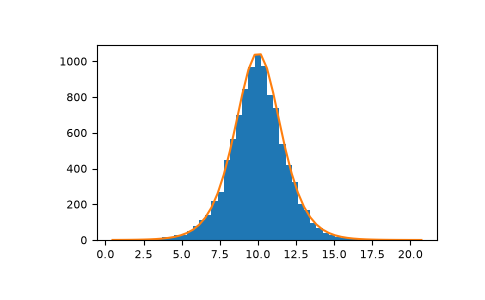# numpy.random.RandomState.logistic¶

method

random.RandomState.logistic(loc=0.0, scale=1.0, size=None)

Draw samples from a logistic distribution.

Samples are drawn from a logistic distribution with specified parameters, loc (location or mean, also median), and scale (>0).

Note

New code should use the logistic method of a default_rng() instance instead; please see the Quick Start.

Parameters
locfloat or array_like of floats, optional

Parameter of the distribution. Default is 0.

scalefloat or array_like of floats, optional

Parameter of the distribution. Must be non-negative. Default is 1.

sizeint or tuple of ints, optional

Output shape. If the given shape is, e.g., (m, n, k), then m * n * k samples are drawn. If size is None (default), a single value is returned if loc and scale are both scalars. Otherwise, np.broadcast(loc, scale).size samples are drawn.

Returns
outndarray or scalar

Drawn samples from the parameterized logistic distribution.

scipy.stats.logistic

probability density function, distribution or cumulative density function, etc.

Generator.logistic

which should be used for new code.

Notes

The probability density for the Logistic distribution iswhere= location and= scale.

The Logistic distribution is used in Extreme Value problems where it can act as a mixture of Gumbel distributions, in Epidemiology, and by the World Chess Federation (FIDE) where it is used in the Elo ranking system, assuming the performance of each player is a logistically distributed random variable.

References

1

Reiss, R.-D. and Thomas M. (2001), “Statistical Analysis of Extreme Values, from Insurance, Finance, Hydrology and Other Fields,” Birkhauser Verlag, Basel, pp 132-133.

2

Weisstein, Eric W. “Logistic Distribution.” From MathWorld–A Wolfram Web Resource. http://mathworld.wolfram.com/LogisticDistribution.html

3

Wikipedia, “Logistic-distribution”, https://en.wikipedia.org/wiki/Logistic_distribution

Examples

Draw samples from the distribution:

>>> loc, scale = 10, 1
>>> s = np.random.logistic(loc, scale, 10000)
>>> import matplotlib.pyplot as plt
>>> count, bins, ignored = plt.hist(s, bins=50)


# plot against distribution

>>> def logist(x, loc, scale):
...     return np.exp((loc-x)/scale)/(scale*(1+np.exp((loc-x)/scale))**2)
>>> lgst_val = logist(bins, loc, scale)
>>> plt.plot(bins, lgst_val * count.max() / lgst_val.max())
>>> plt.show()